# Dividing a decimal by a whole number

##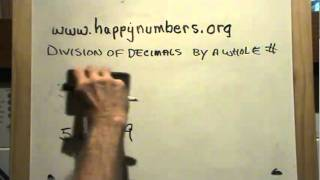By B Barnett

Learn how to divide decimals by whole numbers.# Dividing whole numbers by unit fractions

##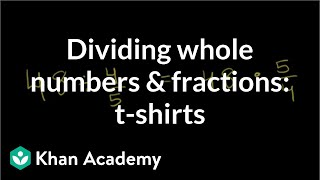By Khan Academy

Dividing a whole number by a fraction? Turn the tables and multiply by the reciprocal of the fraction. Boom!# Dividing whole numbers by unit fractions

##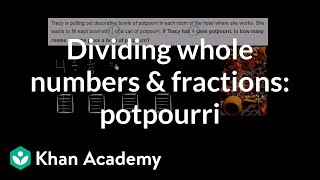By Khan Academy

Solve a word problem by dividing a whole number by a fraction. The example used in this video is 4 ï¿½ï¿½ï¿½ï¿½ï¿½ï¿½ï¿½ï¿½ï¿½ï¿½ï¿½ï¿½ 1/5.# Dividing unit fractions by whole numbers

##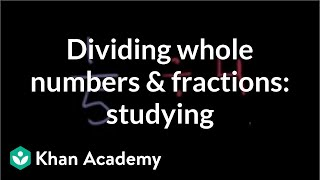By Khan Academy

Solve a word problem by dividing a fraction by a whole number. The example used in this video is 1/5 ï¿½ï¿½ï¿½ï¿½ï¿½ï¿½ï¿½ï¿½ï¿½ï¿½ï¿½ï¿½ 4.# Dividing whole numbers by unit fractions

##By Khan Academy

Dividing a whole number by a fraction? Turn the tables and multiply by the reciprocal of the fraction. Boom!# Dividing whole numbers by unit fractions

##By Khan Academy

Dividing a whole number by a fraction? Turn the tables and multiply by the reciprocal of the fraction. Boom!# Dividing whole numbers by unit fractions

##By Khan Academy

Dividing a whole number by a fraction? Turn the tables and multiply by the reciprocal of the fraction. Boom!# Dividing a whole number by a fraction

##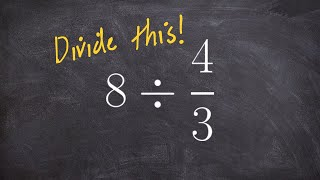By Brian Mclogan

To divide two fractions, we multiply the first fraction by the reciprocal of the second fraction.# Dividing Whole Numbers and Applications 4

##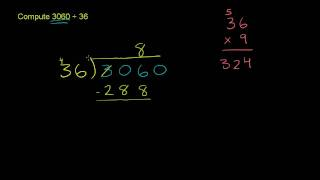By Khan Academy

This video lecture series on Developmental Math by Khan Academy provides developmental math examples from the Monterey Institute. These start pretty basic and would prepare a student for the Worked Examples in Algebra course lectures.....# Dividing unit fractions by whole numbers

##By Khan Academy

Solve a word problem by dividing a fraction by a whole number. The example used in this video is 1/5 ï¿½ï¿½ï¿½ï¿½ï¿½ï¿½ï¿½ï¿½ï¿½ï¿½ï¿½ï¿½ 4.# Dividing unit fractions by whole numbers

##By Khan Academy

Solve a word problem by dividing a fraction by a whole number. The example used in this video is 1/5 ï¿½ï¿½ï¿½ï¿½ï¿½ï¿½ï¿½ï¿½ï¿½ï¿½ï¿½ï¿½ 4.# Dividing whole numbers by unit fractions

##By Khan Academy

Solve a word problem by dividing a whole number by a fraction. The example used in this video is 4 ï¿½ï¿½ï¿½ï¿½ï¿½ï¿½ï¿½ï¿½ï¿½ï¿½ï¿½ï¿½ 1/5.# Dividing unit fractions by whole numbers

##By Khan Academy

Solve a word problem by dividing a fraction by a whole number. The example used in this video is 1/5 ï¿½ï¿½ï¿½ï¿½ï¿½ï¿½ï¿½ï¿½ï¿½ï¿½ï¿½ï¿½ 4.# Dividing whole numbers by unit fractions

##By Khan Academy

Solve a word problem by dividing a whole number by a fraction. The example used in this video is 4 ï¿½ï¿½ï¿½ï¿½ï¿½ï¿½ï¿½ï¿½ï¿½ï¿½ï¿½ï¿½ 1/5.# Dividing whole numbers by unit fractions

##By Khan Academy

Solve a word problem by dividing a whole number by a fraction. The example used in this video is 4 ï¿½ï¿½ï¿½ï¿½ï¿½ï¿½ï¿½ï¿½ï¿½ï¿½ï¿½ï¿½ 1/5.# Dividing decimals 2

##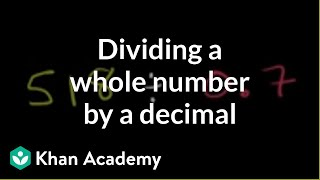By Khan Academy

When dividing a whole number by a decimal, you're going to need to change that decimal into a whole number. How? We'll show you.# 5.NF.B.7C.a. Fraction Divided by Whole Number

##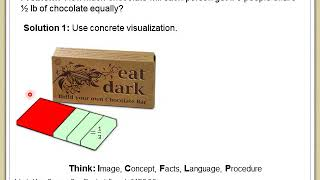By LANL MSA# Dividing whole numbers and fractions: t-shirts

##By Khan Academy# Dividing decimals 2

##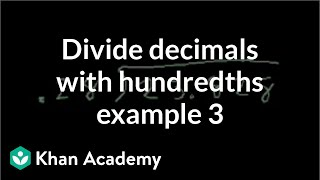By Khan Academy

The old saying is practice makes perfect. Let's keep practicing dividing decimals and making our divisor a whole number.# Dividing decimals 4

##By Khan Academy

The old saying is practice makes perfect. Let's keep practicing dividing decimals and making our divisor a whole number.